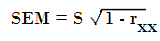# Standard Error of Measurement (SEm): Definition, Meaning

Statistics Definitions > Standard Error of Measurement (SEM)

## What is the Standard Error of Measurement?

The standard error of measurement (SEm) is a measure of how much measured test scores are spread around a “true” score. The SEm is especially meaningful to a test taker because it applies to a single score and it uses the same units as the test.

The SEm is more formally defined as:

“…the standard deviation of errors of measurement that is associated with the test scores for a specified group of test takers ~ AERA, APA, & NCME (1985).”

## SEm Formula

The formula is:Where rxx is the reliability or precision of the test. You’ll sometimes know the reliability of a test, but if you need to calculate it, the formula for rxx is:
rxx = S2T / S2X
Where:

• S2T = variance of the true scores.
• S2X = variance of the observed scores.

Example
An IQ test has a reliability of .7. What is the SEm for the test?
Solution: SEm = 15(&sqrt;(1-.7)) = 15*.548 = 8.22.

## Confidence Intervals

The SEm is usually accompanied by a confidence interval, or a range around the estimated “true” score. The measurement unit is the same as the original test scores. For example, if you’re measuring in points, the SEm will be in points and if you’re measuring in percentages, the SEm will be in percentages. Common SEm confidence intervals and their formulas:
68%CI = Score ±SEM
95%CI = Score ±(1.96*SEM)
99%CI = Score ±(2.58*SEM)

Example: a person scores 100 on a test with an SEm of 2. What is the 68% confidence interval for the spread of scores?
Solution:An SEm of 2 would be one SEM either side of the true score (i.e. between -1 and 1 SEm). Using the formula:
68%CI = Score ±SEM
(100 – 2) = 98
(100 + 2) = 102.
The person’s true score lies between 98 and 102.
What is the 95% confidence interval for the same data?
Using the formula for the 95% CI gives a range from 96.08 to 103.92:
95%CI = Score ±(1.96*SEM) = 100 ± (1.96*2) = 96.08 / 103.92.

## More on Standard Error of Measurement and Reliability

Standard Error of Measurement is directly related to a test’s reliability: The larger the SEm, the lower the test’s reliability.

• If test reliability = 0, the SEM will equal the standard deviation of the observed test scores.
• If test reliability = 1.00, the SEM is zero.

## Standard error of estimation (SEest) a

Standard error of estimation (SEest) is another form of SEm used in tests like the Wechsler Intelligence Scale for Children, 4th Edition (WISC-IV). The SEest takes into account that scores closer to the mean are likely to be more accurate than extreme values. The WISC-IV manual provides a table to interpret theses scores, which are unevenly dispersed and therefore difficult for even professionals to calculate.

Caution: Despite the similarity in names, SEM is not the same as the Standard Error of the Estimate (most commonly just called the Standard Error) or the Standard Error of the mean.
References:
AERA, APA, & NCME (1985). Standards for educational and psychological testing. Washington, D. C.: American Psychological Association. p. 94.

CITE THIS AS:
Stephanie Glen. "Standard Error of Measurement (SEm): Definition, Meaning" From StatisticsHowTo.com: Elementary Statistics for the rest of us! https://www.statisticshowto.com/standard-error-of-measurement/
---------------------------------------------------------------------------Need help with a homework or test question? With Chegg Study, you can get step-by-step solutions to your questions from an expert in the field. Your first 30 minutes with a Chegg tutor is free!